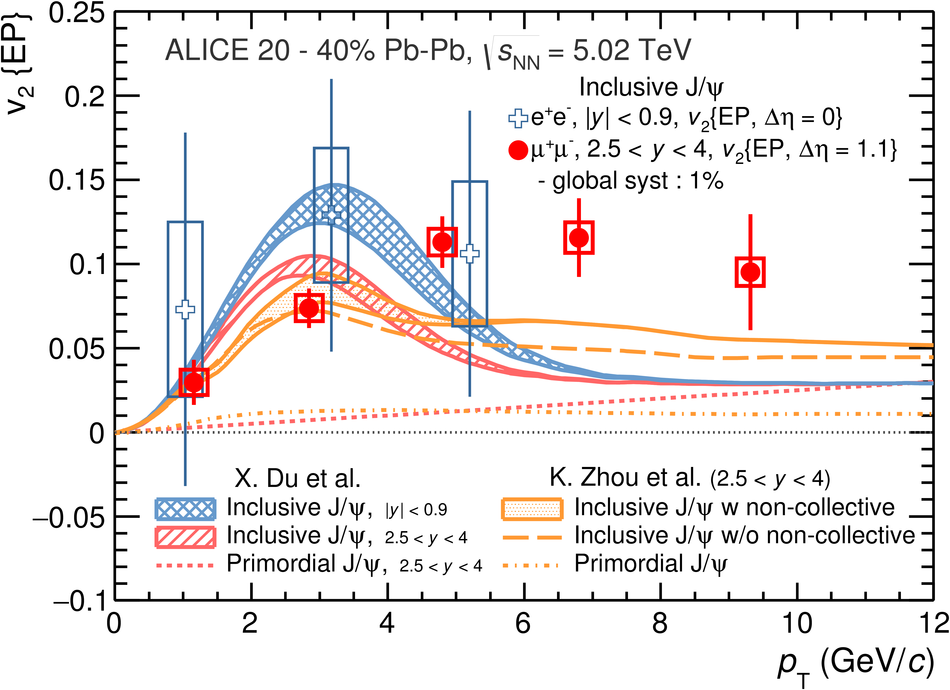# J/$ψ$ elliptic flow in Pb-Pb collisions at $\mathbf{\sqrt{s_{\rm NN}}}$ = 5.02 TeV

We report a precise measurement of the J/$\psi$ elliptic flow in Pb-Pb collisions at $\sqrt{s_{\rm NN}} = 5.02$ TeV with the ALICE detector at the LHC. The J/$\psi$ mesons are reconstructed at mid-rapidity ($|y| <~ 0.9$) in the dielectron decay channel and at forward rapidity ($2.5<~y<~4.0$) in the dimuon channel, both down to zero transverse momentum. At forward rapidity, the elliptic flow $v_2$ of the J/$\psi$ is studied as a function of transverse momentum and centrality. A positive $v_2$ is observed in the transverse momentum range $2 <~ p_{\rm T} <~ 8$ GeV/$c$ in the three centrality classes studied and confirms with higher statistics our earlier results at $\sqrt{s_{\rm NN}} = 2.76$ TeV in semi-central collisions. At mid-rapidity, the J/$\psi$ $v_2$ is investigated as a function of transverse momentum in semi-central collisions and found to be in agreement with the measurements at forward rapidity. These results are compared to transport model calculations. The comparison supports the idea that at low $p_{\rm T}$ the elliptic flow of the J/$\psi$ originates from the thermalization of charm quarks in the deconfined medium, but suggests that additional mechanisms might be missing in the models.

Figures

## Figure 1

 Invariant mass distribution (top) and $\langle \cos 2 (\varphi - \Psi) \rangle$ as a function of $m_{\ell\ell}$ (bottom) of opposite-sign dimuons (left) with $2 < p_{\rm T}< 4$ GeV/$c$ and $2.5 < y < 4$ and dielectrons (right) with $2 < p_{\rm T} < 6$ GeV/$c$ and $|y| < 0.9$, in semi-central (20--40\%) Pb-Pb collisions.## Figure 2

 Inclusive \j \vpt\ at forward and mid-rapidity for semi-central (20--40\%) \pb collisions at $\snn = 5.02$ TeV (see text for details on uncertainties) Calculations from transports model by~ and ~ are also shown (see text for details).## Figure 3

 Inclusive \j \vpt\ at forward rapidity in \pb collisions at $\snn = 5.02$ TeV for three centrality classes, 5--20\%, 20--40\%, and 40--60\%. The average of ${\rm D^0}$, ${\rm D^+}$ and ${\rm D^{*+}}$ \vpt\ at mid-y in the centrality class 30--50\% is also shown for comparison~.